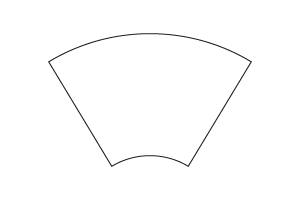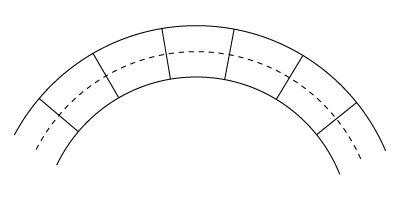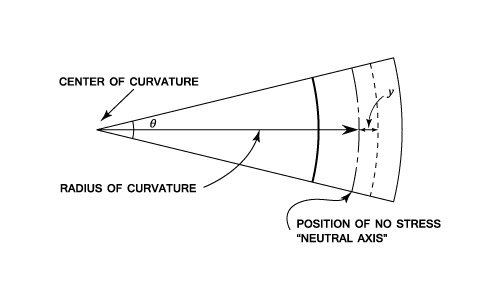•http://facebook.com/
•https://www.google.com/accounts/o8/id
•https://me.yahoo.com

# Bending Stress

An analysis of the simple theory of bending, including moments of area(inertia).

## Bending Theory

If a length of beam is acted upon by a constant bending moment (zero shear force), the stress set up on any cross section must constitute a pure couple equal and opposite to the bending moment. Hence, it can be deduced that one part of the cross section is in tension whilst the other part is in compression. It is clear that for an initially straight beam the inside edge will be in compression and the outside in tension. There will be an intermediate surface where the stress will be zero and this is called the neutral axis.

A beam is a horizontal structural element that is capable of withstanding load primarily by resisting bending. The bending force induced into the material of the beam as a result of the external loads, own weight, span and external reactions to these loads is called a bending moment.

In the following analysis it has been assumed that:
• 1) The material is homogeneous, isotropic and has the same value for Young's Modulus in both tension and compression.
• 2) The beam is initially straight and all longitudinal filaments bend into circular arcs with a common centre of curvature.
• 3) Transverse cross-sections remain plane and perpendicular to the neutral surface after bending.
• 4) The radius of curvature is large compared to the dimensions of the cross section.
• 5) The stress is purely longitudinal and local effects near concentrated loads are neglected.
• 6) The stresses are within the elastic limit of the material and no permanent distortion of the material takes place.

The above diagram shows a beam which is subject to a bending force. If the beam is to bend, there is only one way in which it can happen. If we examine a small bit under stress, it will look like this:Of course it can still be joined up to the sections next to it because the plane sections remain plane.Now consider a small section of the beam under a bending stress.Note that all distances are measured from the "Neutral Axis".

Consider what has happened to an element of the beam which is a distance from the neutral axis. From the above diagram it can be seen that the length has been "stretched" so the strain at from the neutral axis is given by:

Young's Modulus is the ratio of a simple tension stress applied to a material, to the resulting strain parallel to the tension. Also known as modulus of elasticity.

Strain = (Length - Original length)/ (Original length)

But Stress / Strain = (Young's Modulus)

From this it is apparent that is constant for any given bending moment. Stress is directly proportional to the distance from the Neutral Axis. Therefore in the interests of both weight reduction and economy, material is concentrated at the greatest possible distance from the neutral axis. Hence the universal adoption of section steel girders.

If is an element of cross section at a distance from the neutral axis , then for pure bending the net normal force on the cross section must be zero.

Thus, or

This is the condition that passes through the centroid of the section.

Taking moments about the Neutral axis:

This is usually written in terms of , where and is called the moment of inertia of the section or second moment of area. Hence,

Equations (1) and (2) can now be combined into:

Where,
• is the stress at a distance from the Neutral Axis,
• is the Bending Moment,
• is the Moment of area (sometimes called Moment of Inertia),
• is Young's Modulus,
• is the radius of Curvature.